This book is archived and will be removed July 6, 2022. Please use the updated version.

Capacitance

# 52 Capacitor with a Dielectric

### Learning Objectives

By the end of this section, you will be able to:

• Describe the effects a dielectric in a capacitor has on capacitance and other properties
• Calculate the capacitance of a capacitor containing a dielectric

As we discussed earlier, an insulating material placed between the plates of a capacitor is called a dielectric. Inserting a dielectric between the plates of a capacitor affects its capacitance. To see why, let’s consider an experiment described in (Figure). Initially, a capacitor with capacitance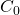when there is air between its plates is charged by a battery to voltage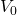. When the capacitor is fully charged, the battery is disconnected. A charge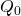then resides on the plates, and the potential difference between the plates is measured to be. Now, suppose we insert a dielectric that totally fills the gap between the plates. If we monitor the voltage, we find that the voltmeter reading has dropped to a smaller value V. We write this new voltage value as a fraction of the original voltage, with a positive number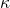,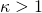: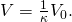The constantin this equation is called the dielectric constant of the material between the plates, and its value is characteristic for the material. A detailed explanation for why the dielectric reduces the voltage is given in the next section. Different materials have different dielectric constants (a table of values for typical materials is provided in the next section). Once the battery becomes disconnected, there is no path for a charge to flow to the battery from the capacitor plates. Hence, the insertion of the dielectric has no effect on the charge on the plate, which remains at a value of. Therefore, we find that the capacitance of the capacitor with a dielectric is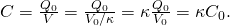This equation tells us that the capacitanceof an empty (vacuum) capacitor can be increased by a factor ofwhen we insert a dielectric material to completely fill the space between its plates. Note that (Figure) can also be used for an empty capacitor by setting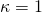. In other words, we can say that the dielectric constant of the vacuum is 1, which is a reference value.

(a) When fully charged, a vacuum capacitor has a voltageand charge(the charges remain on plate’s inner surfaces; the schematic indicates the sign of charge on each plate). (b) In step 1, the battery is disconnected. Then, in step 2, a dielectric (that is electrically neutral) is inserted into the charged capacitor. When the voltage across the capacitor is now measured, it is found that the voltage value has decreased to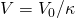. The schematic indicates the sign of the induced charge that is now present on the surfaces of the dielectric material between the plates.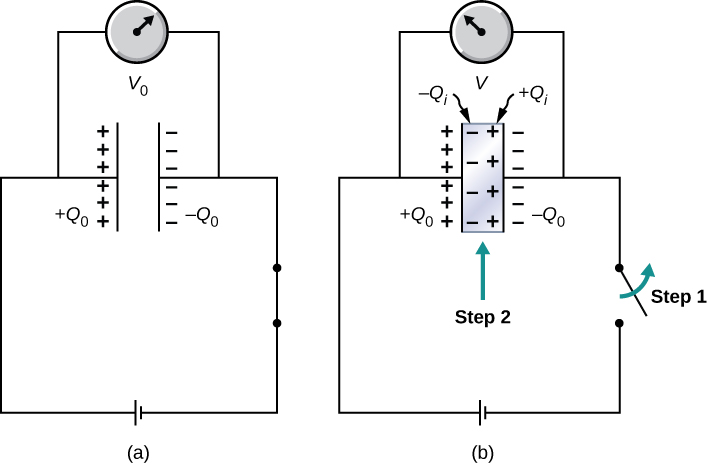The principle expressed by (Figure) is widely used in the construction industry ((Figure)). Metal plates in an electronic stud finder act effectively as a capacitor. You place a stud finder with its flat side on the wall and move it continually in the horizontal direction. When the finder moves over a wooden stud, the capacitance of its plates changes, because wood has a different dielectric constant than a gypsum wall. This change triggers a signal in a circuit, and thus the stud is detected.

An electronic stud finder is used to detect wooden studs behind drywall. (credit top: modification of work by Jane Whitney)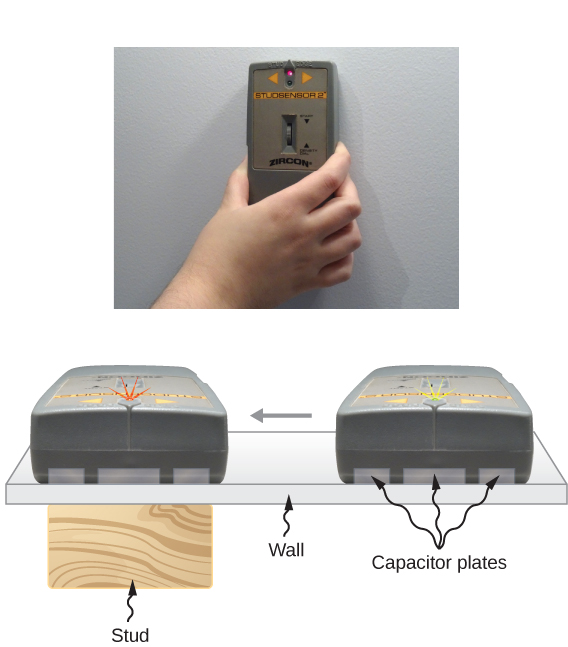The electrical energy stored by a capacitor is also affected by the presence of a dielectric. When the energy stored in an empty capacitor is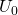, the energy U stored in a capacitor with a dielectric is smaller by a factor of,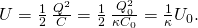As a dielectric material sample is brought near an empty charged capacitor, the sample reacts to the electrical field of the charges on the capacitor plates. Just as we learned in Electric Charges and Fields on electrostatics, there will be the induced charges on the surface of the sample; however, they are not free charges like in a conductor, because a perfect insulator does not have freely moving charges. These induced charges on the dielectric surface are of an opposite sign to the free charges on the plates of the capacitor, and so they are attracted by the free charges on the plates. Consequently, the dielectric is “pulled” into the gap, and the work to polarize the dielectric material between the plates is done at the expense of the stored electrical energy, which is reduced, in accordance with (Figure).

Inserting a Dielectric into an Isolated Capacitor An empty 20.0-pF capacitor is charged to a potential difference of 40.0 V. The charging battery is then disconnected, and a piece of Teflon™ with a dielectric constant of 2.1 is inserted to completely fill the space between the capacitor plates (see (Figure)). What are the values of (a) the capacitance, (b) the charge of the plate, (c) the potential difference between the plates, and (d) the energy stored in the capacitor with and without dielectric?

Strategy We identify the original capacitance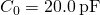and the original potential difference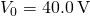between the plates. We combine (Figure) with other relations involving capacitance and substitute.

Solution

1. The capacitance increases to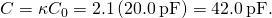2. Without dielectric, the charge on the plates is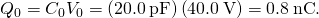Since the battery is disconnected before the dielectric is inserted, the plate charge is unaffected by the dielectric and remains at 0.8 nC.

3. With the dielectric, the potential difference becomes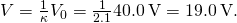4. The stored energy without the dielectric is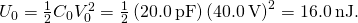With the dielectric inserted, we use (Figure) to find that the stored energy decreases to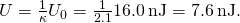Significance Notice that the effect of a dielectric on the capacitance of a capacitor is a drastic increase of its capacitance. This effect is far more profound than a mere change in the geometry of a capacitor.

Check Your Understanding When a dielectric is inserted into an isolated and charged capacitor, the stored energy decreases to 33% of its original value. (a) What is the dielectric constant? (b) How does the capacitance change?

a. 3.0; b.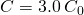### Summary

• The capacitance of an empty capacitor is increased by a factor ofwhen the space between its plates is completely filled by a dielectric with dielectric constant.
• Each dielectric material has its specific dielectric constant.
• The energy stored in an empty isolated capacitor is decreased by a factor ofwhen the space between its plates is completely filled with a dielectric with dielectric constant.

### Conceptual Questions

Discuss what would happen if a conducting slab rather than a dielectric were inserted into the gap between the capacitor plates.

Discuss how the energy stored in an empty but charged capacitor changes when a dielectric is inserted if (a) the capacitor is isolated so that its charge does not change; (b) the capacitor remains connected to a battery so that the potential difference between its plates does not change.

### Problems

Show that for a given dielectric material, the maximum energy a parallel-plate capacitor can store is directly proportional to the volume of dielectric.

An air-filled capacitor is made from two flat parallel plates 1.0 mm apart. The inside area of each plate is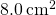. (a) What is the capacitance of this set of plates? (b) If the region between the plates is filled with a material whose dielectric constant is 6.0, what is the new capacitance?

a. 7.1 pF; b. 42 pF

A capacitor is made from two concentric spheres, one with radius 5.00 cm, the other with radius 8.00 cm. (a) What is the capacitance of this set of conductors? (b) If the region between the conductors is filled with a material whose dielectric constant is 6.00, what is the capacitance of the system?

A parallel-plate capacitor has charge of magnitude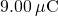on each plate and capacitance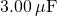when there is air between the plates. The plates are separated by 2.00 mm. With the charge on the plates kept constant, a dielectric with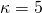is inserted between the plates, completely filling the volume between the plates. (a) What is the potential difference between the plates of the capacitor, before and after the dielectric has been inserted? (b) What is the electrical field at the point midway between the plates before and after the dielectric is inserted?

a. before 3.00 V; after 0.600 V; b. before 1500 V/m; after 300 V/m

Some cell walls in the human body have a layer of negative charge on the inside surface. Suppose that the surface charge densities are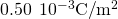, the cell wall is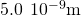thick, and the cell wall material has a dielectric constant of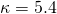. (a) Find the magnitude of the electric field in the wall between two charge layers. (b) Find the potential difference between the inside and the outside of the cell. Which is at higher potential? (c) A typical cell in the human body has volume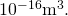Estimate the total electrical field energy stored in the wall of a cell of this size when assuming that the cell is spherical. (Hint: Calculate the volume of the cell wall.)

A parallel-plate capacitor with only air between its plates is charged by connecting the capacitor to a battery. The capacitor is then disconnected from the battery, without any of the charge leaving the plates. (a) A voltmeter reads 45.0 V when placed across the capacitor. When a dielectric is inserted between the plates, completely filling the space, the voltmeter reads 11.5 V. What is the dielectric constant of the material? (b) What will the voltmeter read if the dielectric is now pulled away out so it fills only one-third of the space between the plates?

a. 3.91; b. 22.8 V

### Glossary

dielectric constant
factor by which capacitance increases when a dielectric is inserted between the plates of a capacitor